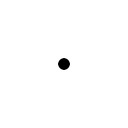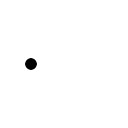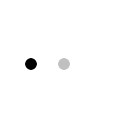# Merge Two Images

• Hi,
my code generate two quadaratic grayscale images. The background is white. In the first image an object is in the center, in the second picture the object is shifted horizontally, as you can see in the figures below.

First Image Second ImageI want to merge these images, so that the second image lies over the first. But with the result that you still see the object from the first image as shown below.

Merged Image:Unfortunately, I'm not able to generate the merged image. So I have to merge the two images.

I tried the following:

``````QImage image1; // converted from the grayscale image to ARGB32 format
QImage image2; // converted from the grayscale image to ARGB32 format

QPainter painter(&image1);
painter.setCompositionMode(); // I tried a few modes but without expected result
painter.drawImage(image1.rect(), image2);
``````

• Hi,

Since you are generating these image, can you generate a translucent background rather than a white one ? That would simplify things to merge the two images.

• @SGaist said in Merge Two Images:

Since you are generating these image, can you generate a translucent background rather than a white one ?

By now: no!

The images are generated with a convolution of a target image and a impulse response function (point spread function, psf). Which is the multiplication of the frequencies in the frequency domain of the two images. To calculate the frequency domain with a FFT the target and the psf are both grayscale (PGM) images.Maybe there is a way to calcuate the FFT of an image with a transparent background, I don't know.

• Then maybe replace white with the alpha channel - e.g. with QImage::createMaskFromColor()

• Thanks for the help.

I solved my problem by converting the gray levels into alpha values.

``````double opacity = 0.5;
int alpha = opacity * (255 - grayValue(x, y));

QImage converted;
converted.setPixelColor(x, y, QColor(0, 0, 0, alpha)
``````

So the whiter the pixel the lower the alpha value. With this converted image I'm able to draw it over the other with

``````QPainter painter(&image1);
painter.drawImage(image1.rect(), converted);
``````